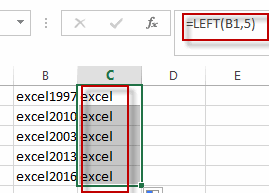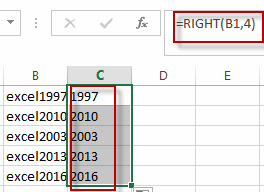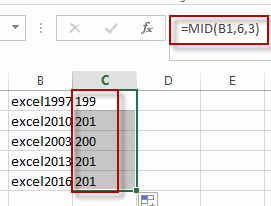# Extract Part of Text String

This post will guide you how to extract part of text string from a cell in Excel. How do I extract a word from a text string in Excel. How to extract a substring from a text string in a Cell in Excel. How to extract a substring from the most left of a text string in Excel. How to extract a word from the most right of a text string in Excel.

Assuming that you have a list of data in the range of cells B1:B5, and you want to get the most left 5 characters from cells, or you want to get the most right 4 characters as a substring from cells, how to achieve it. Let’s see the below detailed steps.

## Extract Word from the Left of a String

To extract word from the left of a text string in a cell in Excel, you can create a formula based on the LEFT function. Like this:

`=LEFT(B1,5)`

Type this formula into a blank cell, then press Enter key in your keyboard. And drag the AutoFill Handle over to other cells to apply this formula.

Let’s see the result:You will see that all the most left 5 characters have been extracted into the range C1:C5.

## Extract Word from the right of a String

If you want to extract a substring from the right of a text string in a cell, you can use a formula based on the RIGHT function. Like this:

`=RIGHT(B1,4)`

Type this formula in a blank cell, Press Enter key to apply this formula. Then drag the AutoFill handle over other cells.## Extract Word from the Mid of a String

If you want to extract a substring from the mid of a text string in a cell, you can use a formula based on the MID function. Like this:

`=MID(B1,6,3)`

This formula will extract a substring from a text string in Cell B1, and the starting position is 6, and the length of substring is 3.### Related Functions

• Excel LEFT function
The Excel LEFT function returns a substring (a specified number of the characters) from a text string, starting from the leftmost character.The LEFT function is a build-in function in Microsoft Excel and it is categorized as a Text Function.The syntax of the LEFT function is as below:= LEFT(text,[num_chars])…t)…
• Excel RIGHT function
The Excel RIGHT function returns a substring (a specified number of the characters) from a text string, starting from the rightmost character.The syntax of the RIGHT function is as below:= RIGHT (text,[num_chars])…
• Excel MID function
The Excel MID function returns a substring from a text string at the position that you specify.The syntax of the MID function is as below:= MID (text, start_num, num_chars)…
Related Posts

Extract substring In Excel

This post will guide you how to use Excel's MID function is a quick and easy way to extract pieces from your text. Use the Excel formula to extract a substring with MID. Note: If you want to extract just ...

How to Split Cells by the First Space in Texts in Excel

Sometimes we may use space to separate texts to different groups in one cell, if we want to split this cell to multiple columns refer to space, we can implement this via ‘Text to Columns’ feature. But is there any ...

How to Convert Uppercase to Lowercase Except the First Letter in Excel

In excel, words can be entered in uppercase, lowercase or mixed. If we want to convert all uppercase to lowercase (except the first letter in some situations), we can use formula with some letter convert related functions to convert them ...

How to Convert Text to Time in Excel

This post will guide you how to convert time string to time in Excel. Assuming that you have a list of text string in your worksheet, and you wish to convert these time string to standard time format, how to ...

How to Remove Last or Trailing Commas in Excel

This post will guide you how to remove trailing commas from cell in Excel. How do I remove comma character at the end of a cell with a formula in Excel 2013/2016. Remove Trailing Commas if you want to remove ...

How to Convert mmddyy to Date in Excel

This post will guide you how to convert non-standard date formats or text to a standard date in Excel. If you have a date with mmddyy format, and you want to change it as the normal date format as mm/dd/yyyy ...

How to Convert Military Time to Standard Time in Excel

This post will guide you how to convert military time to standard time with a formula in Excel. How do I convert from military time 17:29:30 to regular time 5:29:30PM in Excel.  How do I Convert Standard time to military ...

How to Sort Data by Last Character in Excel

This post will guide you how to sort cells by last character in Excel. How do I sort data by the last character with a formula in Excel. How to sort cells based on the last character with User Defined ...

How to Remove the First/Last Word from Text string in Cell

This post will guide you how to remove the first and the last word from a text string in cells in Excel. How do I use a formula to remove first and last word of a text string in Excel. ...

How to Remove Prefix and Suffix in Given Cells in Excel

This post will guide you how to remove suffix form a range of cells in Excel. How do I remove prefix from text string in cells with a formula in Excel. Remove Prefix of Cells Remove Suffix of Cells Assuming ...

Sidebar